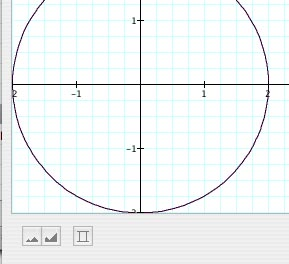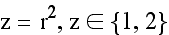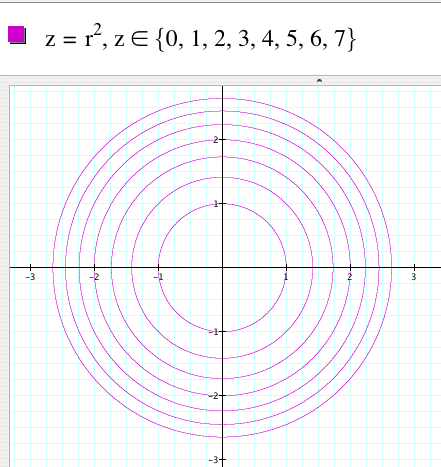Create a contour map of a mathematical function

Material

Graphing Calculator (available from Pacific Tech http://www.pacifict.com/Products.html for \$60 for teachers and \$40 for students.)
Many other mathematical programs will produce contour maps of functions.

Assembly

Enter the equation for the function for which you wish to create a contour map.

for example z = r^2

Simple technique

Under the graph menu choose contour plot. graph>contour plot.

Use the controls at the bottom to control the scale of the plot and the spacing of the contours.Experiment until you get the pattern you like.

You can enter the exact values of the contours you like by typing the equation followed by a comma and z

z = r^2, z

Then press the option key and = at the same time to get the "is an element of the set" symbol. Then type the values of z for which you would like the function plotted between brackets.The program will plot the contour lines.You can scale these contours up and down in size using a graphics editing program or a copier that can enlarge or reduce images.

You can also plot contour maps of functions with the more expensive programs:

Matlab: Contour Plots in Matlab

Mathematica Contour Plots in mathematica

Free Applets

Applet1

 Scientific Explorations with Paul Doherty © 2007 11 November 2007